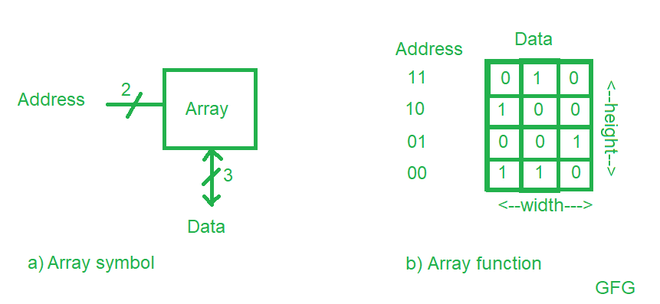# Size of ROM for n-bit Adder/Subtractor

• Difficulty Level : Easy
• Last Updated : 15 Feb, 2021

ROM is a read-only memory which is used to store the data. The memory in the ROM is organized as a two-dimensional array of memory cells. The memory will read or write the contents of one of the rows of the array. This row is specified by an Address which are bits. The value read or written is called Data.Figure a) shows a memory array with two address bits and three data bits. The two address bits specify one of the four rows (data words) in the array. Each data word is three bits wide.
Figure b) shows some possible contents of the memory array.

An array of memory cells with n-bit addresses and m-bit data will haverows and m columns as shown in the figure. Each row of data is called a word. Thus, the array contains aword x m -bit array.

In the case of figure, the array of memory cells with 2-bit addresses and 3-bit Data will haverows which are 4 and 3 columns, so thus the array containsx m =x 3 = 4-word x 3 -bit array.

To get clear understanding let’s take 10 address bits and 32 Data bits then the size of the ROM would be –

`2^(10) x 32 = 1024 x 32 = 32 Kb`

Now to find the size of the ROM we need to find the number of inputs and output bits –

Number of inputs = n(A) + n(B) + 1(Cin) = 2n+1 address bits

Number of outputs = n(sum/diff) + 1(Cout) = n+1

Thus, this would require a 2^(2n+1) x (n+1) -bit ROM.

Here, n(A) means the number of inputs for A and n(B) means the number of inputs for B.

Example-1 : Size of the ROM you could use to program for 16-bit adder/subtractor with Cin and Cout is ____?

Solution :

Number of inputs = 16 (A) + 16 (B) + 1(Cin) = 2(16)+1 = 33 address bits.

Number of outputs = 16 (sum/diff) + 1(Cout) = 16+1 = 17

`Thus, this would require a 2^(2n+1)x(n+1) = 2^(33)x17 bit ROM.`

Example-2 : Size of the ROM you could use to program for 8-bit adder/subtractor with Cin and Cout is ____?

Solution :

Number of inputs = 8 (A) + 8 (B) + 1(Cin) = 2(8)+1 = 17 address bits.

Number of outputs = 8 (sum/diff) + 1(Cout) = 8+1 = 9

`Thus, this would require a 2^(2n+1)x(n+1) = 2^(17)x9 bit ROM.`
My Personal Notes arrow_drop_up